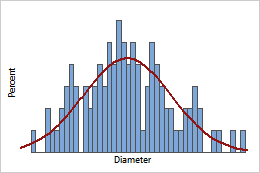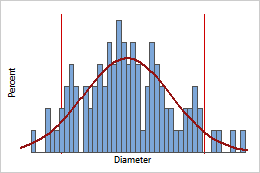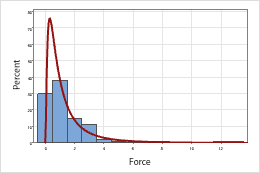# Using the probability density function (PDF)

## What is the probability density function (PDF)?

The probability density function helps identify regions of higher and lower probabilities for values of a random variable.

## Example of a discrete PDF

For a discrete variable, the PDF gives the probability values for given x-values. For example, a candy manufacturer produces a single type of candy in multiple colors. 30% of the candies produced are yellow, 10% are orange, 10% are red, 20% are green, and 30% are blue.Discrete PDFThis bar chart displays the PDF for candy color. Each bar represents the probability of candies of that color expressed as a percentage.

## Example of a continuous PDF

The probability density function (PDF) is an equation that represents the probability distribution of a continuous random variable. For example, a machine that cuts corks for wine bottles produces corks with different diameters. In the following bar chart of cork diameters, each bar represents the percent of corks with that corresponding diameter.Continuous PDFThe curve is the PDF for cork diameter. Use the PDF to identify regions of higher and lower probabilities for values of a random variable. For example, only a small percentage of corks (1%) have a diameter less than 2.8 cm.Continuous PDF with specification limitsIf the specification limits for cork diameter are 2.85 cm to 3.15 cm, the PDF can indicate the probability density values of all corks from this process that meet the specifications.
The shape of the PDF is different for different distributions. The familiar bell-shaped curve represents the PDF for a normal distribution. While cork diameter follows a normal distribution, other measurements, such as the force it takes to pull the cork out of the wine bottle, may follow a different distribution. For example, the PDF for a lognormal distribution has a long right tail.Lognormal PDFBecause a wine bottle occasionally requires an unusual amount of force to remove the cork, measures of this force often follow a distribution with a long right tail such as the lognormal distribution.
By using this site you agree to the use of cookies for analytics and personalized content.  Read our policy